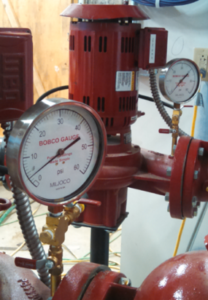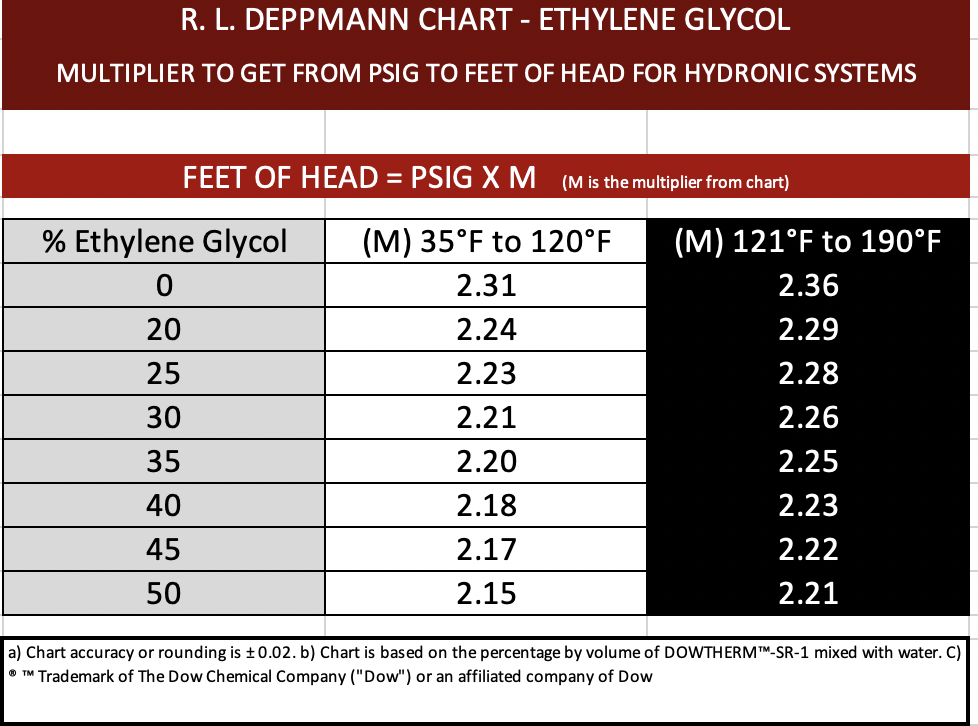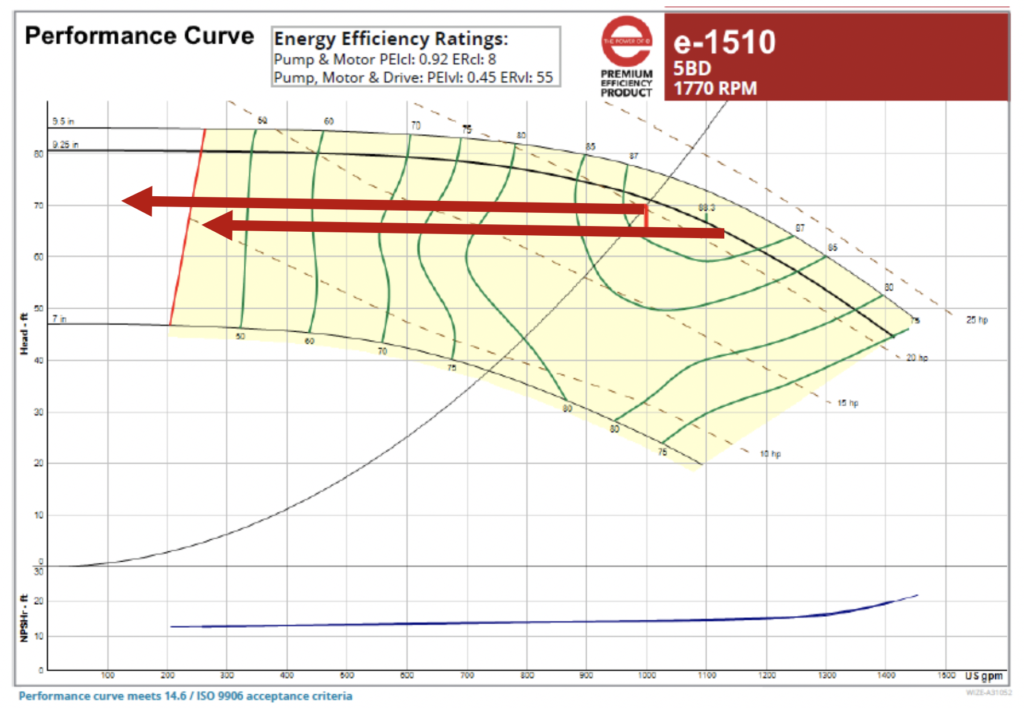# Centrifugal Pump Gauge Readout Corrections for Hydronic Glycol Systems

Norm Hall
/
November 4, 2019Ask any test/balance or service technician in the hydronic HVAC world to convert PSIG to feet of head and the multiplier of 2.31 is the immediate response. It is one of the simple formulas we learn early in our careers. There are times when that number changes enough to make a difference and hydronic glycol systems are one of those times.

The formula for the conversion of gauge readings to feet of head is:

FEET = (PSIG X 2.31)/SPECIFIC GRAVITY

Specific gravity is normally close to one for water but not so in glycol systems. Here are some helpful tables I made for your use. Let’s look at the tables and then I’ll explain how much of a difference they can make.## How much of a difference can it make in a test and balance report?

Last week, the R. L. Deppmann Monday Morning Minutes explained Velocity Head Correction when reading out pumping systems. Sometimes it makes a difference and sometimes the difference is so small it is negligible. This specific gravity correction is similar. Let’s look at an example curve for 1000 GPM at 70 feet from the Bell & Gossett ESP-Systemwize program. Assume the gauge differential was 30 PSIG. So, 30 x 2.31 = 69.3 which looks close to our 70 feet of design. If the fluid was 50% propylene glycol at 40°F the formula would be 30 X 2.18 = 65.4.This simple example shows the balance report would be off by 15%. This could make a difference. If the fluid was the same 50% fluid at 170°F the answer would be 67.5’ head. Still a difference…. but not as much of a difference.

Engineers, balance technicians, service contractors and pump representatives should understand the effect of specific gravity and know when to use these handy tables for hydronic glycol systems, from the R. L. Deppmann Monday Morning Minutes.